# Direction Sense MCQ - 3

## 20 Questions MCQ Test Reasoning Aptitude for Competitive Examinations | Direction Sense MCQ - 3

Description
Attempt Direction Sense MCQ - 3 | 20 questions in 15 minutes | Mock test for LR preparation | Free important questions MCQ to study Reasoning Aptitude for Competitive Examinations for LR Exam | Download free PDF with solutions
QUESTION: 1

### A person going 40m to the south of his house.Then he turned left and go 20m and turn to north and walk 30m then start walking to my house. In which direction he was walking now ?

Solution: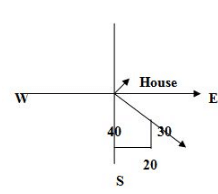QUESTION: 2

### A walked 20m towards south and then took a left turn and walked 40m. Again he took left turn and walked 15m. Finally he turned right and walked 10m and stopped. Q. How far is he from starting point ?

Solution: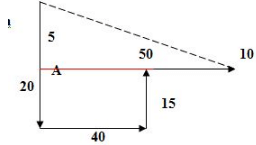QUESTION: 3

### A walked 20m towards south and then took a left turn and walked 40m. Again he took left turn and walked 15m. Finally he turned right and walked 10m and stopped. Q. In which direction A is now facing

Solution:
QUESTION: 4

Which of the following point is exactly 5m away from point E ?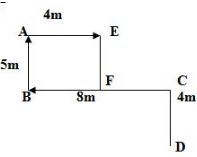Solution:clearly, F point is verically downward in figure from point E at a distance of 5 m

QUESTION: 5

In which direction is point D with respect to point A ?Solution:
QUESTION: 6

If P is 45m northeast of Q and R is 45m northwest of Q then R is in which direction with respect to P ?

Solution: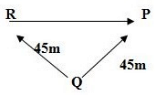QUESTION: 7

Direction: Point A is 9m towards the east of point B. Point C is 5m towards the south of point A. Point D is 3m towards the west of point C. Point E is 5m towards the north of point D. Point F is 7m towards the south of point D.
Which of the following points are in a straight line ?

Solution: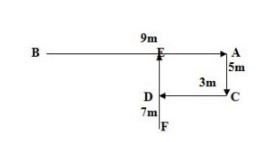QUESTION: 8

​

Direction: Point A is 9m towards the east of point B. Point C is 5m towards the south of point A. Point D is 3m towards the west of point C. Point E is 5m towards the north of point D. Point F is 7m towards the south of point D.
Which of the following points are in a straight line ?

Q.

What is E position with respect to F ?

Solution:
QUESTION: 9

Direction: Point A is 9m towards the east of point B. Point C is 5m towards the south of point A. Point D is 3m towards the west of point C. Point E is 5m towards the north of point D. Point F is 7m towards the south of point D.
Which of the following points are in a straight line ?

Q.

A man walked 15m towards South, took a left turn and walked 20m again he took a left turn and walked 15m. How far he from the starting point ?

Solution:
QUESTION: 10

Direction: Point A is 9m towards the east of point B. Point C is 5m towards the south of point A. Point D is 3m towards the west of point C. Point E is 5m towards the north of point D. Point F is 7m towards the south of point D.
Which of the following points are in a straight line ?

Q.

Kumar walks 8km towards North. From there he walks 4 km towards south. Then, he walks 3km towards East. How far and in which direction is he with reference to his starting point ?

Solution: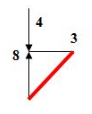QUESTION: 11

Direction: Point A is 9m towards the east of point B. Point C is 5m towards the south of point A. Point D is 3m towards the west of point C. Point E is 5m towards the north of point D. Point F is 7m towards the south of point D.
Which of the following points are in a straight line ?

Q.

A boy goes 6 m North and turns east and walk 8 m. Again he turns to his right and walk 4 m. After this he turns to his right and walks 8 m. How far is he from his starting point ?

Solution: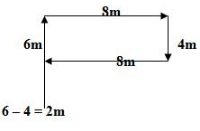QUESTION: 12

Direction: Point A is 9m towards the east of point B. Point C is 5m towards the south of point A. Point D is 3m towards the west of point C. Point E is 5m towards the north of point D. Point F is 7m towards the south of point D.
Which of the following points are in a straight line ?

Q.

Aruna starts from a point and walks 2m east and then turns left and walks 4 km and turns right and walks 6m again then she starts towards a point 4m towards her right, from where again  she  walks  2 m left. How far is she from the starting point?

Solution: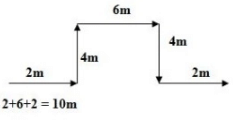QUESTION: 13

Direction: Point A is 9m towards the east of point B. Point C is 5m towards the south of point A. Point D is 3m towards the west of point C. Point E is 5m towards the north of point D. Point F is 7m towards the south of point D.
Which of the following points are in a straight line ?

Q.

A lady walks 7 metres toward west, then turns left and walks 7 metres. She  again turns left and walks 7 metres. She takes a 135 degree turn rightwards and walks straight. In which direction is she walking now?

Solution: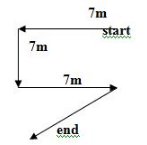QUESTION: 14

There are 5 shops A, B, C, D and E. B is to the northeast of E. D is 2km to the east of E, who is 6km to the west of A. C is to the northwest of D and in the line of EB. D is 4km the south of B.

Q.

What is the distance between town D and town A ?

Solution:QUESTION: 15

There are 5 shops A, B, C, D and E. B is to the northeast of E. D is 2km to the east of E, who is 6km to the west of A. C is to the northwest of D and in the line of EB. D is 4km the south of B.

Q.

In which direction is A with respect to B ?

Solution:
QUESTION: 16

There are 5 shops A, B, C, D and E. B is to the northeast of E. D is 2km to the east of E, who is 6km to the west of A. C is to the northwest of D and in the line of EB. D is 4km the south of B.

Q.

In which direction is C with respect to A ?

Solution:
QUESTION: 17

A man walked 5m towards north, took a left turn and walked for 10m. He then took a right turn and walked for 20m and again took right turn and walked 10m. How far he is from starting point ?

Solution: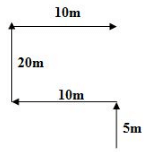QUESTION: 18

Arvind walked 25m towards west, took a left turn and walked 15m. He again took a left turn and walked 30m. He then took a right turn and stopped. Now he was facing which direction ?

Solution: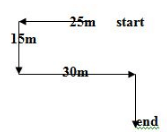QUESTION: 19

Selva moves towards South–east a distance of 6 m, then she moves towards West and travels a distance of 10 m. From here, she moves towards North-west a distance of 6 m and finally she moves a distance of 2 m towards East and stood at that point. How far is the starting point from where she stood?

Solution: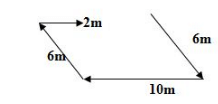QUESTION: 20

P, Q, R and S are playing cards. P and Q are partners. S faces towards North. If P faces towards West, then who faces towards East ?

Solution: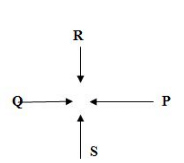Use Code STAYHOME200 and get INR 200 additional OFF Use Coupon Code